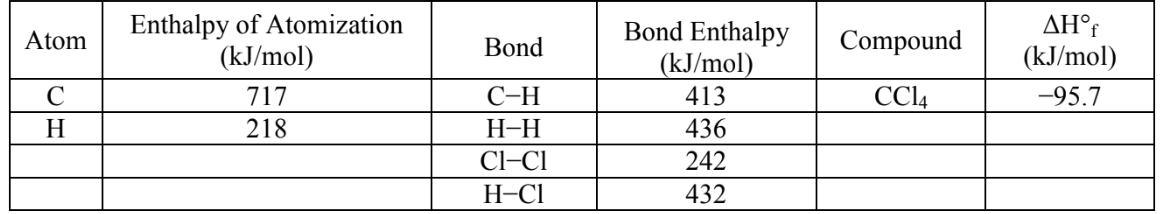# Problem: Estimate ΔH°rxn for the following reaction, using the chemical reactions given above and the ΔH°rxn values you calculated in part (a). Show all work to support your answer and circle your final answer.1⁄4 CH4 (g) + Cl2 (g) → 1⁄4 CCl4 (l) + HCl (g)           ΔH°rxn = ?a. +85.8 kJb. +226 kJc. +301 kJd. -667 kJe. -266 kJ

###### FREE Expert Solution

For this problem, we have to calculate the enthalpy of reaction (ΔH) using the given bond enthalpies.

1⁄4 CH4 (g) + Cl2 (g) → 1⁄4 CCl4 (l) + HCl (g)

Recall that the change of enthalpy for a chemical reaction (ΔH) can be calculated using bond energies:

To determine the enthalpy of reaction, we need to first see what bonds are present in the reactants and the products.

94% (3 ratings)###### Problem DetailsEstimate ΔH°rxn for the following reaction, using the chemical reactions given above and the ΔH°rxn values you calculated in part (a). Show all work to support your answer and circle your final answer.

1⁄4 CH4 (g) + Cl2 (g) → 1⁄4 CCl4 (l) + HCl (g)           ΔH°rxn = ?

a. +85.8 kJ

b. +226 kJ

c. +301 kJ

d. -667 kJ

e. -266 kJ